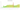# С̲

## Kyrgyzstani som

### Kyrgystani som

The som is Kyrgyzstan's designated currency.

#### how has the value of the currency changed in the last year?## USD 1 = KGS 87.59

How much is10 US dollars worth inKyrgystani soms?
At the current exchange rate, 10 US dollars is worth 875.9 Kyrgystani soms
How much is50 US dollars worth inKyrgystani soms?
At the current exchange rate, 50 US dollars is worth 4,379.5 Kyrgystani soms
How much is100 US dollars worth inKyrgystani soms?
At the current exchange rate, 100 US dollars is worth 8,759 Kyrgystani soms
How much is500 US dollars worth inKyrgystani soms?
At the current exchange rate, 500 US dollars is worth 43,795 Kyrgystani soms
How much is2,000 US dollars worth inKyrgystani soms?
At the current exchange rate, 2,000 US dollars is worth 175,180 Kyrgystani soms
How much is10 US dollars worth inKyrgystani soms?
At the current exchange rate, 10 US dollars is worth 875.9 Kyrgystani soms
How much is50 US dollars worth inKyrgystani soms?
At the current exchange rate, 50 US dollars is worth 4,379.5 Kyrgystani soms
How much is100 US dollars worth inKyrgystani soms?
At the current exchange rate, 100 US dollars is worth 8,759 Kyrgystani soms
How much is500 US dollars worth inKyrgystani soms?
At the current exchange rate, 500 US dollars is worth 43,795 Kyrgystani soms
How much is2,000 US dollars worth inKyrgystani soms?
At the current exchange rate, 2,000 US dollars is worth 175,180 Kyrgystani soms